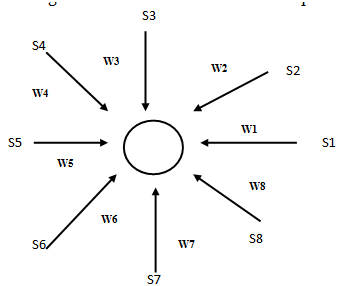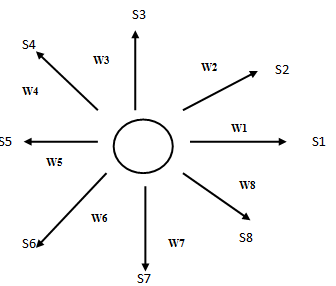### Competitive Learning

In the networks with competitive and corporative learning, it can be said that the neurons compete and cooperate ones with the others to give a specific task. Differing from the net of Hebbian learning basis, where many output nodes can be activated simultaneously, in the case of competitive learning only one can be activated at a time. In this method , the neurons of the output  layer compete among themselves to become active. This feature is highly suited to discover the statistical features of a set of input patterns.
Three elements of competitive learning
A set of neurons are same except for the randomly distributed weights. There fore each neuron responds differently to a given input.
A limit is imposed on the strength of each neuron.
A mechanism that permits the neuron to compete so that only one is active at a time. The neuron that wins is called winner-take-all-neuron.

In its simplest form the network has a single layer of output neurons each of which is fully connected to the input (source) nodes. The network may include feedback connections among the neurons. The feedback connections perform lateral inhibition. Each neuron tends to inhibit the other neuron to which it is laterally connected.
For an output neuron k to be the winning neuron, its activation input Xk  for a specified input pattern S must be the largest among all the neurons in the output layer. The output layer is therefore set to 1; and the output of all other neurons that lose the competition are set to zero.

1         if    Xk> Xl for all l ≠ k
Yk =
0        otherwise

Where the induced local field Xk  represents the combined  action for all the feed forward and feedback inputs to neuron k.  The weights Wkj for all input nodes j connected to output node k are +ve and distributed such that
å Wkj  =1 for all k

The neuron then learns by shifting its weights from its inactive inputs to active inputs. According the standard competitive learning rule, the change in weight DWkj  is defined by
h (Sj - Wjk         if neuron k wins the competition
DWkj  =        0             if neuron k loses the competition

Where h is the learning parameter. This rule has the overall effect of moving the weight vector Wk of the winning neuron k of the toward the input pattern S.

Instar and Outstar
The instar and outstar can be connected together to form complex networks.
Instar Learning :- An star configuration consists of a neuron fed by a set of inputs through synaptic weights. It can be trained to respond to a specific input vector S. Training is accomplished by adjusting its weights so that the weight vector becomes similar to the input
vector.The output is calculated as the weighted sum of its inputs or the dot product of the weight vector with  the input vector. The dot product of normalized vectors is a measure of similarity between the vectors. Once the training is over the output from the is maximum(the neuron fires) when the input vector is similar to the weight vector.
 The weight update equation is given as

Wji(m+1)= Wji(m)+h[Si(m)-Wji(m)] The value of h starts with 0.1 and is generally reduced during the training process.
Outstar Learning
In outstar configuration, the neuron drives a set of synapses through its synaptic weights. The outstar produces a desired excitation pattern to other neurons whenever it fires. The weight update equation isWkj(m+1)= Wkj(m)+b[Yk(m)-Wkj(m)]
b- is learning parameter . The value of b is close to 1 at the beginning and is gradually reduced during training.